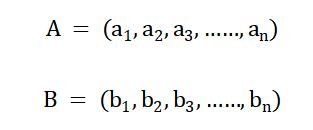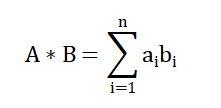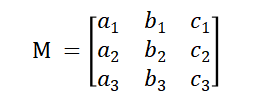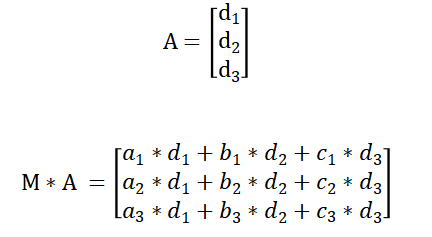1506字，阅读需时6分钟1.2*10+1.8*6+2.1*5=33.3

A=(1.2,1.8,2.1)

B=(10,6,5)

A和B是三维向量，向量的这种表示方式称为行向量，向量分量书写在同一行上。向量也可以使用下面的方式来表示：1.2*10+1.8*6+2.1*5=33.2A*B称为向量A与B的点积。

```import numpy as np
# 定义向量A和B
A = np.array([1.2,1.8,2.1])
B = np.array([10,6,5])
# 计算向量A和B的点积
result = np.dot(A,B)
print("%.2f" % (result))```

（a）A*B = B * A

（b）A*(B+C) = A*B + A*C

（c）c(A*B)=(cA)*B=A*(cB)

（d）A*A > 0，若A!=0

（e）A*A=0，若A=0

Gray = R*0.299 + G*0.587 + B*0.114```import numpy as np
import matplotlib.pyplot as plt
import matplotlib.image as mpimg

def rgb2gray(rgb):
# 矩阵与向量的点积运算
return np.dot(rgb, [0.299, 0.587, 0.114])